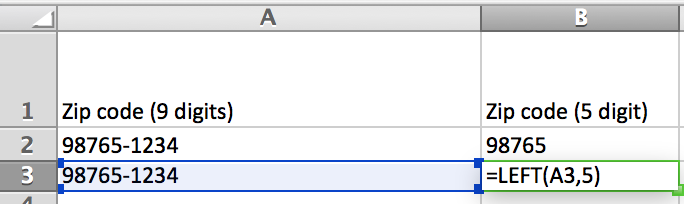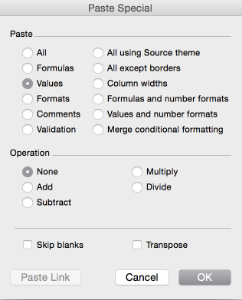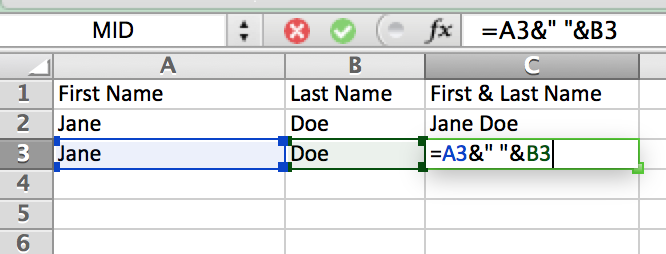# How to Clean Data in Excel: 5 Simple Tricks

One of the biggest hurdles in working with data is when the data is “dirty”. Typically dirty data is when you have duplicates, names that have been spelled differently across data entries, or your data is in the wrong format. Cleaning up your data helps make sure that everything is categorized correctly so you can make better sense of it. Here are five easy steps you can take to clean your data using Excel.

## #1. Search and Replace

One of the best tricks to clean up data is the Search/Replace function in Excel. The feature allows you to find a specific word and Replace it with a new one. This is particularly useful when different spelling variations appear for the same word such as ‘non-profit’ and ‘nonprofit’ or ‘California’ and ‘CA’.

You can find this function under Edit -> Replace. Alternatively you can use the keyboard shortcut Control+F (Command+F on Mac) and click ‘Replace’.

Simply type in the word you are looking to replace in the top entry field and the word you want to replace it with in the bottom entry field and click ‘Replace All’. That’s how easy it is.

Notice you can select whether you want to replace entries only in the sheet you have selected or across the entire workbook. You can also select a range of cells and only have the function replace the words in that range.

Bonus tip: The Replace function is also an easy way to delete extra spaces in cells. Just add a space in the top entry field and nothing in the bottom entry field and click ‘Replace all’, all extra spaces in your selected range will be deleted.

## #2. LEFT/RIGHT/MID

The LEFT/RIGHT/MID formulas allow you to select a cell from which you would only like certain parts of the content. One example could be that zip codes have been entered with 9 digits, e.g. 12345-6789, but you only need the first five digits. To make this conversion, insert a column next to the zip code column and use the LEFT formula to extract the first five digits.

Excel formula:
=LEFT(text, [num_chars])

‘Text’ refers to the cell you want to convert and [num_chars] is the number of characters you want to pull from the cell. The formula starts from the left of the selected cell and pulls the number of characters you want, in this case the number of characters is 5, as you only want the first five digits.Turn your 9 digit zipcode entry into a 5 digit zip code with the LEFT formula.

Once you have entered the formula for all cells, it's important you turn your formulas into plain text. Highlight the cells that contain the LEFT formula, copy the cells (CONTROL+C or COMMAND+C on Mac computers), go to Edit -> Paste Special. Now, select 'Values' and press 'OK'. This will paste the cells as plain text so the formula no longer appears in the cells. This allows you to move cells around without having to worry about the formulas being wrong.Paste Special in Excel. You can find this function under Edit -> Paste Special.

The RIGHT formula works the same way as the LEFT formula, except it starts from the right and counts backwards. Everything else is the same. The MID formula on the other hand is slightly different. MID is short for Middle, which means you can capture parts of a word, starting at any point in a cell.

As an example, say you have a phone number with a country code, such as this one: 001 (206) 123 4567, but you are only interested in capturing the area codes. i.e. the '206' part. The MID formula can help you extract this part.

Excel formula:
=MID(text,start_num,num_chars)

=MID([select a cell],[where you want to start counting from],[the number of characters you want to capture])

In the example of the phone 001 (206) 123 4567 where you want to capture the area code 206, you want to start at the number 2 after the first parenthesis and capture 3 characters. All characters and spaces are allocated a number starting with 1. In this case the first 0 is character number 1, the next 0 is character number 2, and so on. If you count 001 (206) 123 4567, the area code starts with character number 6 (notice, spaces also count as a character). The formula will therefore become:

Excel formula:
=MID(A1,6,3).

## #3. Combining Text

If you have two cells that you would like to combine, say ‘First name’ and ‘Last name’. There is a neat function within Excel to do this. By using the ampersand (&) you can combine content of two cells into one new cell. If you just want to combine two text strings, simply start out with the equal sign, select the first cell add an '&' and select the second cell.

Excel formula
=[Cell 1]&[Cell 2]

However, in this case we want to combine a first name and a last name, say Jane Doe. To avoid that the output becomes JaneDoe, you need to add a space between the two cells. To do this add two quotation marks with a space in-between, and include it as an element between the two cells. The formula should look like this.

Excel formula:
=[Cell 1]&” “&[Cell 2]Easily combine content of two cells using the & in Excel.

## #4. LOWER and UPPER

A way to make data entries more uniform is by using the formula LOWER. LOWER turns all letters of a specific cell into lowercase letters. This is a quick way of making sure all data entries for a category are bucketed together. A common case where this formula comes in handy is with place names, as these tend to be spelled in various ways, e.g. Boston, boston, bOSTN, or BOSTON. With this formula, all the data entries will be spelled boston.

Excel formula:
=LOWER(text)

(the ‘text’ part in the parentheses refers to the cell you want to convert to lowercase letters)

A variation of this formula is UPPER, which works in the same fashion as LOWER but turns all letters into uppercase letters.

As you might have noticed, place names will not necessarily be spelled correctly if you only use one of these formulas, but if you combine these two formulas and the LEFT and MIDDLE formulas, you can makes sure it’s spelled Boston instead of boston or BOSTON.

This is slightly more complicated, so hang tight. Regardless of if Boston is spelled, BOSTON, boston, BostoN, or bOSTON, you would want the first letter to be capitalized (UPPER) and the remaining characters to be lowercase (LOWER). To do this you will have to combine the formulas and functions UPPER, LEFT, '&', UPPER, and MID.

Let's break it down to see what needs to be done.

• #1. Capture the first character 'b'

• #2. Make sure the first character is always capitalized

• #3. Capture everything but the first character i.e. 'oston'

• #4. Make sure everything after the first character is lowercase.

• #5. Combine 'B' with 'oston'

For the purpose of simplicity, let's assume the data entry (bOSTON) is in cell A2, but this could obviously be any cell.

#1 and #2: To capture the first letter we use the LEFT formula, but since this needs to be capitalized we wrap the UPPER formula around it, so it looks like this:

=UPPER(LEFT(A2,1) -> This will return 'B'

#3 and #4: To capture everything but the first character we use the MID formula, and to make sure it's all lowercase, we wrap it in the LOWER formula. It should look like this:

=LOWER(MID(A2,2,60) -> This will return 'oston'

Notice the last part of the formula that says 60 , we could have limited that to 5, which is the number of characters 'oston' amounts to, but if you have a column with a lot of different city names, make sure you capture all characters by having a large number in the latter part of the formula.

#5: To combine all four steps we can use the ampersand trick we learned above. This means the final formula should look like this:

=UPPER(LEFT(A2,1)&LOWER(MID(A2,2,60)

(Notice you only include equal signs once at the beginning when you combine formulas)

## #5. Deleting Duplicates

If you want a dataset with unique rows, you will need to look for duplicates.

First, sort your column alphabetically. Select a column, go to Data -> Sort , then select the column you want to organize and select 'A to Z'.

Now that everything is ordered alphabetically by the column you want to check, you can use the IF formula to highlight duplicates. The way you do it is by comparing one cell to the one above. The IF formula works like this:

Excel formula:
=IF(logical_text, [value_if_true],[value_if_false])

Explained: =IF([logical test],[If logical is true then what?],[If logical test is false then what?])

In our case we want to start by comparing cell C3 to cell C2 to see if they are the same, this is the logical test. If they are the same we want the cell we are using to check for duplicates to write Duplicate (value_if_true) and if they are not the same (value_if_false), the cell should just be blank. The formula will look like this:

Excel formula:
=IF(C3=C2,"Duplicate","")

The quotation marks tells Excel that it should be considered plain text, this still works even though you don't add any text between the quotation marks which is why the cell will appear blank. In the image below, you will see that 'Georg Lucas' is recognized as a duplicate, all the other cells are blank because the logical test is false and thus returns a blank cell.

Your final step is to use the paste special -> values for the 'Check for duplicates' column, then you sort the column alphabetically so all the duplicates congregate before you highlight the rows and delete them.

Voila! You have now cleaned your dataset without spending hours. These are only some of the things you can do, what other tips do you know of that can clean your data? Let us know in the comment section below.